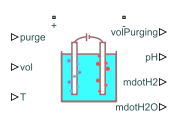# Electrolyzer

Since R2022a

•Libraries:
Simscape / Electrical / Sources

## Description

The Electrolyzer block represents the electric load associated with an electrolyzer. An electrolyzer consists of an anode and a cathode separated by an electrolyte. The block calculates the amount of produced hydrogen based on the electrical energy provided and the water temperature in the tank.

In polymer electrolyte membrane (PEM) electrolyzers, these chemical reactions define the anode and cathode conversions:

`$2{H}_{2}O\to {O}_{2}+4{H}^{+}+4{e}^{-}$`

`$4{H}^{+}+4{e}^{-}\to 2{H}_{2}$`

An electrolysis stack comprises several series-connected individual electrolyzer cells. Specify the Assumption pH parameter to model this block either in a pH-dependent state or in an ideal state with constant pH.

### Equations

The Electrolyzer block calculates the electrical power by using

`${P}_{E}=\frac{{v}^{2}}{{R}_{T}},$`

where v is the voltage and RT is the total resistance. The total resistance, RT, is defined by

`$\frac{1}{{R}_{T}}=\frac{1}{{R}_{el}}+\frac{1}{R},$`

where:

• R is the external resistance.

• ${R}_{el}=\frac{\rho \left(pH\right)x}{A}.$ This value is a resistive term obtained from the cross-sectional transport area, A, the distance anode-cathode, x, and the electrical resistivity of the solution, ρ(pH).

The molar energy stored across the electrical load enables the process of water electrolysis and is calculated by this equation:

`${G}_{E}=\frac{{P}_{E}}{1\text{\hspace{0.17em}}mol/s}.$`

To accomplish electrolysis, the electrolyzer requires a minimum amount of energy

`${G}_{\mathrm{min}}=\Delta H-\frac{T}{{T}_{0}}{T}_{\Delta S},$`

where:

• ΔH is the water enthalpy.

• TΔS is the reaction entropy.

• T is the water temperature.

• T0 is the reference temperature.

If the electrical energy is not sufficient to dissociate the water, the electrolyzer does not produce hydrogen. Therefore, the Electrolyzer block calculates the mole rate of electron, mole, and the mole rate of hydrogen, molH2

`$mo{l}_{e}=\left\{\begin{array}{cc}\frac{\mu \left({G}_{E}-{G}_{\mathrm{min}}\right)}{{e}_{V}{N}_{A}}& {G}_{E}>{G}_{\mathrm{min}}\\ 0& {G}_{E}\le {G}_{\mathrm{min}}\end{array}$`

`$mo{l}_{H2}=\left\{\begin{array}{cc}\frac{{N}_{c}mo{l}_{e}}{2},& {G}_{E}>{G}_{\mathrm{min}}\\ 0,& {G}_{E}\le {G}_{\mathrm{min}}\end{array}$`

where:

• NA is the Avogadro constant.

• eV is the energy per electron.

• Nc is the number of cells.

• μ is the temperature-dependent efficiency of the electrolysis.

The current that flows through the electrolyzer tank is then obtained as

`$i=mo{l}_{e}F,$`

where F is the Faraday constant.

The Electrolyzer block calculates the mass rates of consumed water and generated hydrogen by using these equations:

`$\begin{array}{l}{\stackrel{˙}{m}}_{H2\text{\hspace{0.17em}}O}=mo{l}_{H2}{M}_{H2\text{\hspace{0.17em}}O}\\ {\stackrel{˙}{m}}_{H2}=mo{l}_{H2}{M}_{H2}\end{array}$`

where MH2O is the molar mass of water and MH2 is the molar mass of hydrogen.

To compute the pH of the water in the tank, the block uses

`$pH=-\mathrm{ln}\left(\frac{{x}_{hp}}{mo{l}_{H2_acc}}\right),$`

where:

• ${x}_{hp}=\left\{\begin{array}{cc}\int mo{l}_{e},& pH\text{\hspace{0.17em}}assumed\text{\hspace{0.17em}}constant\\ \int \left(1-purge\right)mo{l}_{e},& pH\text{\hspace{0.17em}}assumed\text{\hspace{0.17em}}dynamic\end{array}$ captures the floating hydrons (H+) in the solution.

• $mo{l}_{H2_acc}=\frac{V{\rho }_{H2\text{\hspace{0.17em}}O}\left(T\right)}{{M}_{H2\text{\hspace{0.17em}}O}}$ is the number of moles of water.

• V is the volume.

• ρH2O(T) is the density of the water in the tank.

The residual protons in the solution inside the electrolyzer naturally increases the pH and conductivity of the water in the tank over time. The increase in conductivity leads to a run-away phenomenon. To prevent the run-away, you must purge the water in the tank by taking out the volume of the tank and fill it with clean water.

## Ports

### Conserving

expand all

Electrical conserving port associated with the anode of the electrolyzer tank.

Electrical conserving port associated with the cathode of the electrolyzer tank.

### Input

expand all

Purge logic input, specified as a physical signal. This signal enables the substitution of the water in the tank.

Water volume in the electrolyzer tank, in m3, specified as a physical signal.

Water temperature, in K, specified as a physical signal.

### Output

expand all

Volume of water consumed for purging, in m3, specified as a physical signal.

Water pH in the electrolyzer tank, specified as a physical signal.

Mass flow rate of the hydrogen, in g/s, specified as a physical signal.

Mass flow rate of the consumed water, in g/s, specified as a physical signal.

## Parameters

expand all

Whether to assume constant or dynamic pH in the electrolyzer tank.

Amount of external resistance.

Normal area to the vector that links the anode and the cathode. Positively charged ions travel across this area from anode to cathode, where they get reduced and the hydrogen is produced.

Distance between the anode and the cathode, submerged in the electrolytic solution.

Number of electrode pairs.

Number of cells in the electrolyzer.

Initial pH value of the water in the tank.

Constant pH value of the water in the tank.

#### Dependencies

To enable this parameter, set Assumption pH to `Constant`.

Vector of pH values. This parameter must have the same number of elements as the Electrical resistivity vector, rho_vec parameter.

#### Dependencies

To enable this parameter, set Assumption pH to `Dynamic`

Vector of electrical resistivity values. This parameter must have the same number of elements as the pH vector, pH_vec parameter.

#### Dependencies

To enable this parameter, set Assumption pH to `Dynamic`

Vector of temperatures for efficiency. This parameter must have the same number of elements as the Electrolysis efficiency vector, efficiency(T) parameter.

Vector of electrolysis efficiency values. This parameter must have the same number of elements as the Temperature vector for efficiency, T_vec parameter.

 Maximilian Schalenbach et al. “A Perspective on Low-Temperature Water Electrolysis - Challenges in Alkaline and Acidic Technology”. International Journal of Electrochemical Science 13, (2018): 1173-1226.